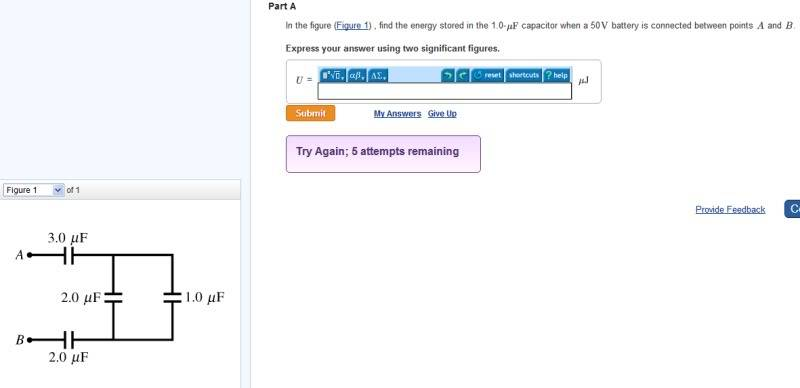# Find stored energy

## Homework Statement## Homework Equations

stored energy = (Capicitance)(Voltage)/2

## The Attempt at a Solution

3+2+2+1 = 8 microfarad or 8 * 10 ^-6 F

Capacitance given to be 1 * 10 ^ -6 F

Voltage is 50 V

J = (8* 10 ^ -6 F) (50 V) ^ 2
J = .01 J

SammyS
Staff Emeritus
Homework Helper
Gold Member

## Homework Statement

[ IMG]http://i33.photobucket.com/albums/d86/warnexus/storedenergy_zps00e9c6b9.jpg[/PLAIN]

## Homework Equations

stored energy = (Capicitance)(Voltage)/2

## The Attempt at a Solution

3+2+2+1 = 8 microfarad or 8 * 10 ^-6 F

Capacitance given to be 1 * 10 ^ -6 F

Voltage is 50 V

J = (8* 10 ^ -6 F) (50 V) ^ 2
J = .01 J
The capacitors are not all in parallel.

To decide which are in series and what they're in series with, and which are in parallel and what they're in parallel with, treat them as if they are resistors.

(I wasn't convinced you knew how to analyze the series/parallel combination of resistors you posted yesterday.)

Which of the capacitors are in parallel ? Which in series. ?

Last edited:
The capacitors are not all in parallel.

To decide which are in series and what they're in series with, and which are in parallel and what they're in parallel with, treat them as if they are resistors.

(I wasn't convinced you knew how to analyze the series/parallel combination of resistors you posted yesterday.)

Which of the capacitors are in parallel ? Which in series. ?

Hi Sammy! I thought parallel circuits take on multiple paths.

i checked the textbook and try to make sense of it and based on their diagram 2 and 1 are in parallel. 3 and 2 are in series

it seems capacitors in series take on one linear path and capacitors in parallel take on multiple path to travel to the next circuit

Last edited:
SammyS
Staff Emeritus
Homework Helper
Gold Member
Hi Sammy! I thought parallel circuits take on multiple paths.

i checked the textbook and try to make sense of it and based on their diagram 2 and 1 are in parallel. 3 and 2 are in series

it seems capacitors in series take on one linear path and capacitors in parallel take on multiple path to travel to the next circuit
Starting at point A ... finishing at point B:

Any current leaving A has to pass through the 3μF capacitor.

Then current comes to the two capacitors on the vertical wires. Current at this part of the circuit (actual any particular charged particle) can through either the 1μF capacitor OR the 2μF capacitor. The current from the 1μF capacitor then rejoins the current from 2μF capacitor.
So these two capacitors are in parallel with each other. You can also think of the circuit as a path. The current goes from having a single path to a choice between two paths, then the paths join back together (without there being other path branching of to somewhere else). That part in parentheses is important in more complicated circuits.

Any current leaving those two capacitors then all must pass through the lower 2μF capacitor before moving on to point B.

What we have is:

The 3μF capacitor is in series with the combination of the two capacitors we earlier identified as being in parallel -- those two being 1μF & 2μF capacitors. That combination is in series with the lower 2μF capacitor.

The first thing to do is to "replace" the parallel capacitors (1μF & 2μF) with a single equivalent capacitor. You then have three capacitors in series.